Default

Default can be used with one two or three arguments, and each form is discussed below.

Default[f]

In the next cell (f) has the default value 0.  Depending on the pattern used f[e] can be treated as f[e,0] or  f[0,e].  If the second argument in the pattern is optional f[e] is treated as f[e,0].  If the first argument in the pattern is optional f[e] is treated as f[0,e].  All throughout this discussion on Default details the functions that are given default values will have no attributes. However, the values assigned to Default[f] have a different effect when (f) has the OneIdentity attribute.  This is covered in the discussion of OneIdentity.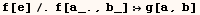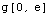Default[f,i]

In the cell below values are given for Default[f,2],  Default[f,3] and definitions for evaluating (f) are given.The definitions for (f) above are used in the input cells below.  For the cells below the default values are not needed because the definitions for (f) could be used directly.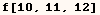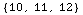Now for the next input (f) has only two arguments, and the definition for integer arguments is used.  However, the definition can only be used if a default value is inserted for the optional argument.  The applicable definition indicates that the second argument is optional.  The default value for the second argument of (f) is 4 so the value 4 is used for the second argument.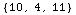Then another input is evaluated where (f) has three arguments,and the definition for rational arguments is used.Again the definition can only be used if a default value is inserted for the optional argument.The applicable definition indicates the third argument is optional.The default value for the third argument of (f) is 1/8 so the value 1/8 is used for the third argument.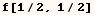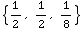In the next cell an attempt to make a rule for (f) with the first argument optional is not permitted.  That's because (f) only has default values for the second and third arguments.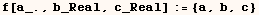Default[f,i,n]

We can also use Default[f,i,n] to specify the default value to use when argument (i) out of (n) arguments is optional.  New default values and definitions for (f) are given below to demonstrate this.  The input below uses definitions for (f) with integer arguments and rational arguments.In each case below the use of a default values were not needed because the definitions for (f) could be used directly.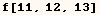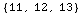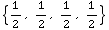Now in the next input (f) has two integer arguments.  The definition can only be used when a default value is inserted for the optional (second) argument.  Once a default value is inserted for the optional argument we will have three arguments.  The default value for the second of three arguments in (f) is 8 so a value of 8 is inserted as the second argument.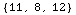Then for another input (f) has three rational arguments.  Again the definition for rational arguments can only be used if a default value is inserted for the optional (second) argument.  Once a value is inserted for the second argument we will have four arguments.  The default value for the second of four arguments for (f) is 1/16 so a value of 1/16 is inserted as the second argument.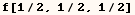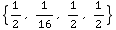In the next cell we can't give a rule where the second of two arguments is optional.  That is not permitted because we don't have a value assigned to Default[f, 2]  or  Default[f, 2, 2].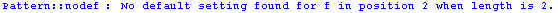Created by Mathematica  (May 16, 2004)

Back to Ted’s Tricks index page